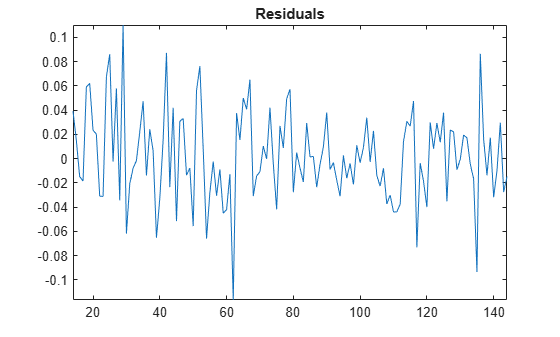Main Content

# Estimate Multiplicative ARIMA Model

This example shows how to estimate a multiplicative seasonal ARIMA model using `estimate`. The time series is monthly international airline passenger numbers from 1949 to 1960.

### Load Data and Specify Model.

Load the airline data set.

```load Data_Airline y = log(Data); T = length(y); Mdl = arima('Constant',0,'D',1,'Seasonality',12,... 'MALags',1,'SMALags',12);```

### Estimate Model.

Use the first 13 observations as presample data, and the remaining 131 observations for estimation.

```y0 = y(1:13); [EstMdl,EstParamCov] = estimate(Mdl,y(14:end),'Y0',y0)```
``` ARIMA(0,1,1) Model Seasonally Integrated with Seasonal MA(12) (Gaussian Distribution): Value StandardError TStatistic PValue _________ _____________ __________ __________ Constant 0 0 NaN NaN MA{1} -0.37716 0.073426 -5.1366 2.7972e-07 SMA{12} -0.57238 0.093933 -6.0935 1.1047e-09 Variance 0.0013887 0.00015242 9.1115 8.1249e-20 ```
```EstMdl = arima with properties: Description: "ARIMA(0,1,1) Model Seasonally Integrated with Seasonal MA(12) (Gaussian Distribution)" Distribution: Name = "Gaussian" P: 13 D: 1 Q: 13 Constant: 0 AR: {} SAR: {} MA: {-0.377161} at lag  SMA: {-0.572379} at lag  Seasonality: 12 Beta: [1×0] Variance: 0.00138874 ```
```EstParamCov = 4×4 0 0 0 0 0 0.0054 -0.0015 -0.0000 0 -0.0015 0.0088 0.0000 0 -0.0000 0.0000 0.0000 ```

The fitted model is

`$\Delta {\Delta }_{12}{y}_{t}=\left(1-0.38L\right)\left(1-0.57{L}^{12}\right){\epsilon }_{t},$`

with innovation variance 0.0014.

Notice that the model constant is not estimated, but remains fixed at zero. There is no corresponding standard error or t statistic for the constant term. The row (and column) in the variance-covariance matrix corresponding to the constant term has all zeros.

### Infer Residuals.

Infer the residuals from the fitted model.

```res = infer(EstMdl,y(14:end),'Y0',y0); figure plot(14:T,res) xlim([0,T]) title('Residuals') axis tight```When you use the first 13 observations as presample data, residuals are available from time 14 onward.

References:

Box, G. E. P., G. M. Jenkins, and G. C. Reinsel. Time Series Analysis: Forecasting and Control. 3rd ed. Englewood Cliffs, NJ: Prentice Hall, 1994.

Download ebook